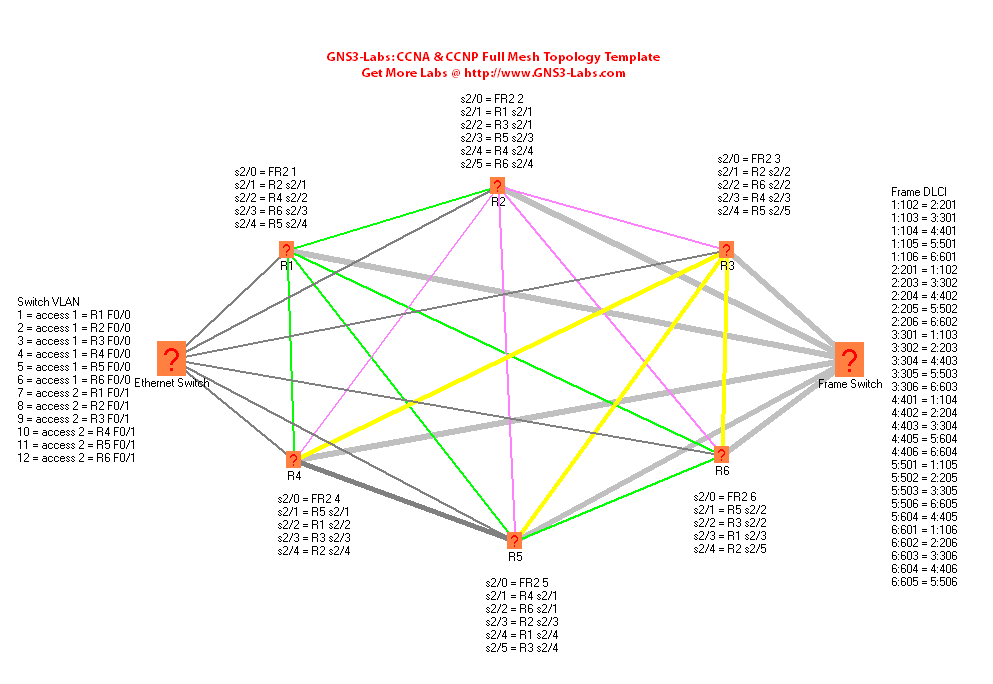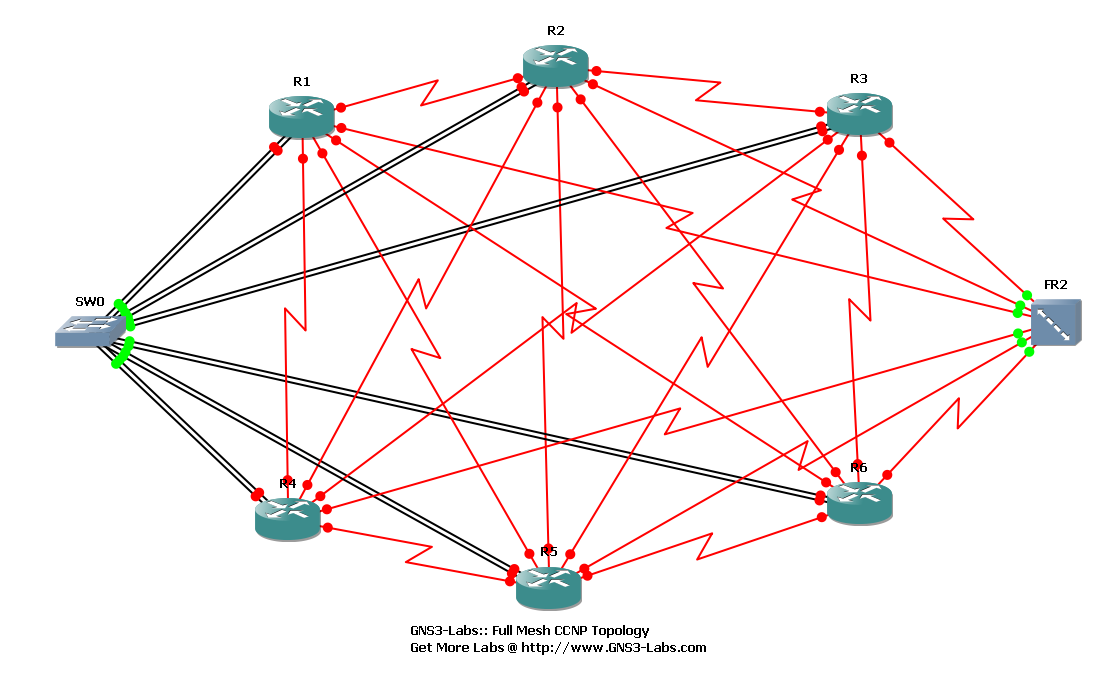# Lab. Dynagen de préparation au CCNA

Voici un Lab de préparation à  la CCNA, créée par un CCIE. Il parait que l’on peut toucher Ã  95% des commandes et protocoles de la CCNA et du CCNP.
Chris, le CCIE explique ce lab. de préparation: « I created one simple topology that seems to handle 95% of my CCNA & CCNP lab requirements. It isn’t fancy, but it should save people an hour+ of labor (that is how long it took me to create this topology).

I was getting tired of making one lab per exercise in the Cisco Lab Portfolios and the Certification Zone lab exercises. So I whipped up a 6 router setup that could handle the majority of the router scenarios I am using for practice.

It consists of 6 7206 routers in a full mesh topology. Each router has 2 ethernet connections to a dynamips â€™switchâ€™, one each in VLAN1 and VLAN2.

Each router has at least 4 frame connections to the other routers in the topology.

Each router also has 1 frame connection to a frame relay switch, with full mesh DLCI between all routers.

I use dynagen, not GNS3. I created a diagram of the topology using the freeware tool called Network Notepad (http://www.networknotepad.com/) so I can keep track of all the different connections.

Some of the CCNA & CCNP stuff calls for using the Cisco SDM. I created one generic_startup.txt config file. It sets the password to cisco for the router, and enables the http server. It also sets the exec-timeout on con0 to infinite, and disables domain lookup (your pings will work quickly now). All you have to do is bridge GNS3 or Dynagen to your physical network card, and you can use the Cisco SDM to interface with the routers. Or SecureACS, the VPN client, VMWare images, etcâ€¦.

Warning: this topo uses a lot of RAM. When I have all 6 routers running, my memory utilization jumps from 900mb to 3.5GB. When all 6 routers are idle, I am seeing 10-15% CPU utilization – I have an Intel Core2 Quad processor. »

Routers Used: 7206

IOS: c7200-advipservicesk9-mz.124-2.T• Voici le .net de cette topologie:

autostart = False
[localhost:7202]
workingdir = C:\Program Files\GNS3\Projects\Router\CCNA-CCNP-Full.Mesh.Topology\CCNA-CCNP-Full.Mesh.Topology-Chris_working
udp = 10200
[]
midplane = std
image = C:\Program Files\GNS3\Images\c7200-jk9o3s-mz.124-17a\C7200-JK.BIN
idlepc = 0x606e8c80
ghostios = True
[[ROUTER R5]]
console = 2004
cnfg = C:\Documents and Settings\Administrator\My Documents\cisco stuff\generic_startup.txt
f0/0 = SW0 5
f0/1 = SW0 11
slot1 = PA-2FE-TX
slot2 = PA-8T
s2/0 = FR2 5
s2/1 = R4 s2/1
s2/2 = R6 s2/1
s2/3 = R2 s2/3
s2/4 = R1 s2/4
s2/5 = R3 s2/4
slot3 = PA-POS-OC3
x = -42.0
y = 217.0
[[ROUTER R6]]
console = 2005
cnfg = C:\Documents and Settings\Administrator\My Documents\cisco stuff\generic_startup.txt
f0/0 = SW0 6
f0/1 = SW0 12
slot1 = PA-2FE-TX
slot2 = PA-8T
s2/0 = FR2 6
s2/1 = R5 s2/2
s2/2 = R3 s2/2
s2/3 = R1 s2/3
s2/4 = R2 s2/5
slot3 = PA-POS-OC3
x = 267.0
y = 133.0
[localhost:7200]
workingdir = C:\Program Files\GNS3\Projects\Router\CCNA-CCNP-Full.Mesh.Topology\CCNA-CCNP-Full.Mesh.Topology-Chris_working
[]
midplane = std
image = C:\Program Files\GNS3\Images\c7200-jk9o3s-mz.124-17a\C7200-JK.BIN
idlepc = 0x606e8c80
ghostios = True
[[ROUTER R1]]
console = 2000
cnfg = C:\Documents and Settings\Administrator\My Documents\cisco stuff\generic_startup.txt
f0/0 = SW0 1
f0/1 = SW0 7
slot1 = PA-2FE-TX
slot2 = PA-8T
s2/0 = FR2 1
s2/1 = R2 s2/1
s2/2 = R4 s2/2
s2/3 = R6 s2/3
s2/4 = R5 s2/4
slot3 = PA-POS-OC3
x = -288.0
y = -251.0
[[ROUTER R2]]
console = 2001
cnfg = C:\Documents and Settings\Administrator\My Documents\cisco stuff\generic_startup.txt
f0/0 = SW0 2
f0/1 = SW0 8
slot1 = PA-2FE-TX
slot2 = PA-8T
s2/0 = FR2 2
s2/1 = R1 s2/1
s2/2 = R3 s2/1
s2/3 = R5 s2/3
s2/4 = R4 s2/4
s2/5 = R6 s2/4
slot3 = PA-POS-OC3
x = -35.0
y = -302.0
[[FRSW FR2]]
1:102 = 2:201
1:103 = 3:301
1:104 = 4:401
1:105 = 5:501
1:106 = 6:601
2:201 = 1:102
2:203 = 3:302
2:204 = 4:402
2:205 = 5:502
2:206 = 6:602
3:301 = 1:103
3:302 = 2:203
3:304 = 4:403
3:305 = 5:503
3:306 = 6:603
4:401 = 1:104
4:402 = 2:204
4:403 = 3:304
4:405 = 5:604
4:406 = 6:604
5:501 = 1:105
5:502 = 2:205
5:503 = 3:305
5:506 = 6:605
5:604 = 4:405
6:601 = 1:106
6:602 = 2:206
6:603 = 3:306
6:604 = 4:406
6:605 = 5:506
x = 470.5
y = -49.5
[[ETHSW SW0]]
1 = access 1
2 = access 1
3 = access 1
4 = access 1
5 = access 1
6 = access 1
7 = access 2
8 = access 2
9 = access 2
10 = access 2
11 = access 2
12 = access 2
x = -501.5
y = -32.0
[localhost:7201]
workingdir = C:\Program Files\GNS3\Projects\Router\CCNA-CCNP-Full.Mesh.Topology\CCNA-CCNP-Full.Mesh.Topology-Chris_working
udp = 10100
[]
midplane = std
image = C:\Program Files\GNS3\Images\c7200-jk9o3s-mz.124-17a\C7200-JK.BIN
idlepc = 0x606e8c80
ghostios = True
[[ROUTER R4]]
console = 2003
cnfg = C:\Documents and Settings\Administrator\My Documents\cisco stuff\generic_startup.txt
f0/0 = SW0 4
f0/1 = SW0 10
slot1 = PA-2FE-TX
slot2 = PA-8T
s2/0 = FR2 4
s2/1 = R5 s2/1
s2/2 = R1 s2/2
s2/3 = R3 s2/3
s2/4 = R2 s2/4
slot3 = PA-POS-OC3
x = -302.0
y = 149.0
[[ROUTER R3]]
console = 2002
cnfg = C:\Documents and Settings\Administrator\My Documents\cisco stuff\generic_startup.txt
f0/0 = SW0 3
f0/1 = SW0 9
slot1 = PA-2FE-TX
slot2 = PA-8T
s2/0 = FR2 3
s2/1 = R2 s2/2
s2/2 = R6 s2/2
s2/3 = R4 s2/3
s2/4 = R5 s2/5
slot3 = PA-POS-OC3
x = 267.0
y = -254.0
[GNS3-DATA]
configs = CCNA-CCNP-Full.Mesh.Topology-Chris_configs
workdir = CCNA-CCNP-Full.Mesh.Topology-Chris_working
[[NOTE 1]]
text = GNS3-Labs:: Full Mesh CCNP Topology\nGet More Labs @ http://www.GNS3-Labs.com
x = -149.0
y = 270.5
rotate = 0

Et voici la configuration basique de ces routeurs:

!
no ip domain lookup
ip http server
!
line vty 0 4
exec-timeout 0 0
!
!
end

Plus d’infos dans quelques jours, quand j’aurai eu le temps de tester ce lab….### Benoit

Network engineer CCIE #47705, focused on R&S, Data Center and SDN.

### One Comment

1.5 juin 2009